﻿ PipeLay > Technical Notes > Technical Note 2 - Strain Computations

# Technical Note 2 - Strain Computations

Navigation:  PipeLay > Technical Notes >

# Technical Note 2 - Strain Computations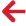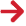## Overview

This technical note describes the calculation of the various strain outputs provided by PipeLay. These comprise bending, hoop, axial and Von Mises strains and the calculation of each of these is now described in turn.

## Bending Strain

The computation of bending strain in PipeLay is based on curvature. The maximum bending strain, εb, at a particular location at a particular time occurs at the outer surface of the cross section and is defined as follows: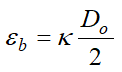(TN.2.1)

where κ is curvature and Do is the effective external diameter.

## Hoop Strain

Hoop strain is computed using a combination of direct strain from the hoop stress and indirect strain from axial and radial stress through Poisson’s ratio or effect. Specifically, the hoop strain, εh, is as follows: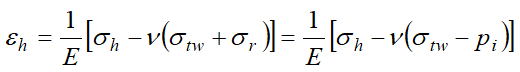(TN.2.2)

where E is Young’s Modulus, σh is hoop stress, ν is Poisson’s ratio, σtw is axial or ‘true wall’ stress, σr is radial stress and pi is internal pressure. Note that this hoop strain value corresponds to that at the inner surface and neutral bending axis. Refer to Technical Note 1 for the derivation of the different stress terms.

## Axial Strain

Axial strain is computed using a combination of direct strain from the axial stress and indirect strain from hoop and radial stress through Poisson’s ratio or effect. Specifically, the axial strain, εa, is as follows: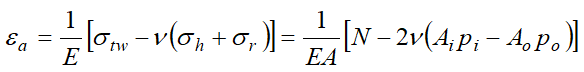(TN.2.3)

where E is Young’s Modulus, σtw is axial or ‘true wall’ stress, σh is hoop stress, σr is radial stress, A is effective cross section area, N is axial force or ‘true wall tension’, ν is Poisson’s ratio, Ai is internal area, pi is internal pressure, Ao is external area, and po is outer pressure. Note that Ao is calculated from the buoyancy diameter Db computed internally by PipeLay, depending on the user specified inputs to the Pipe Section component. Refer to Technical Note 1 for the derivation of the different stress terms.

## Von Mises Strain

The Von Mises strain is computed using a combination of the predicted bending, hoop and axial strains computed using Eqns. (TN.2.1-TN.2.3) above. Specifically, the Von Mises strain, εvm, is as follows: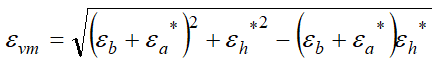(TN.2.4)

where the εb, εa* and εh* are the bending, hoop and axial strains, respectively. Note that the asterisks above the hoop and axial terms indicate that these strains arise from the direct stresses only. In other words, the Poisson related contributions in Equations TN.2.2 and TN.2.3 are ignored in the case of the Von Mises strain calculation with only direct stress generated strain considered.